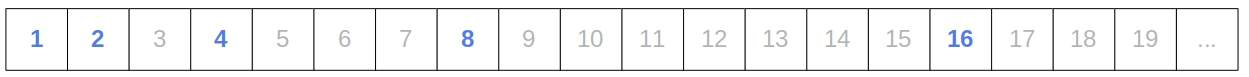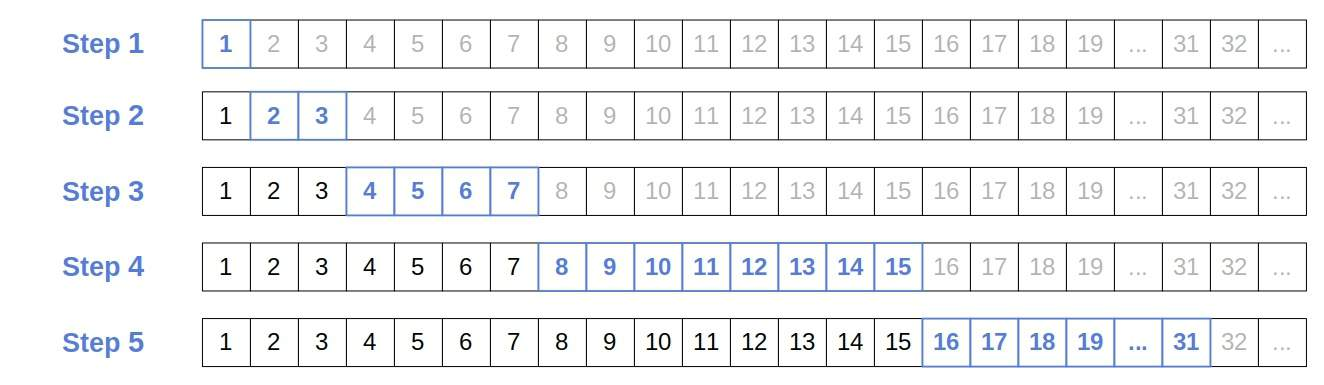## 1. Introduction

In this tutorial, we’ll explain the Exponential Search algorithm.

In a classical search problem, our goal is to find the index of a valuein an-element sorted array(for). For instance, we can use the Binary Search algorithm to look for anyin a logarithmic time.

However, there are cases when Binary Search isn’t fast enough or even possible to use. For example, ifis so large thatcan’t fit into memory and evenis unacceptably high, or if we don’t have an array to search but a functiondefined over an infinite integer domain. In the latter case, we have an infinite collectionto search, so we’re solving an unbounded search problem. In the former case,is technically finite, but since it’s too large for our memory, we deal with it as if it’s unbounded.

We can’t use Binary Search since it assumes that its input is a finite array. One of the methods we can use is the Exponential Search algorithm. It assumes that we can evaluate (or find)for any integerin the search domain and thatis sorted.

Exponential Search has two phases.

The first phase finds the portion ofthat containsif it’s present in the array. More specifically, the first phase finds the numbersuch that.

The idea is to partitioninto sub-arrays of incremental sizes and check them consecutively until we find the one that can contain. The boundary indices of those sub-arrays are powers of 2, hence the algorithm’s name:In the second phase, the algorithm looks forinusing Binary Search.

### 3.1. Pseudocode

Here’s the pseudocode:So, we first check if, which simplifies to testing if. If the test fails, we check if. If that isn’t the case, we test if, then,, and so on until we find the boundaries that capture:.

Afterward, we run Binary Search on. If it doesn’t find, then it isn’t an element of. If it does, it will return the index ofin the sub-array as an integer from 1 to. We adjust it to get the index ofin the entire array by adding the offset(the starting index of the sub-array) and taking away 1.

### 3.2. Example

Let’s say we’re looking for a number at the 17th position in a very long array. By testing the boundaries with the Exponential Search, we locate the portion ofthat contains the number in 5 steps:## 4. Complexity

Ifis the index ofin, the time complexity of the first phase is, as illustrated in the example above.

In the second phase, we run Binary Search on the sub-array whose size is:The time complexity of Binary Search isfor an array of size, so the complexity of the second phase of Exponential Search is:So, the total combined runtime complexity of both phases is.

From there, we see that Exponential Search pays off in the cases whenis very large, and the sought element is near the arrays’ beginning.

## 5. Conclusion

In this article, we presented Exponential Search. It’s a search algorithm we use to find values in unbounded collections like ordered ranges of functions defined over natural numbers. Additionally, we can also use it to search arrays too big to fit into memory.

1 Comment
Inline Feedbacks